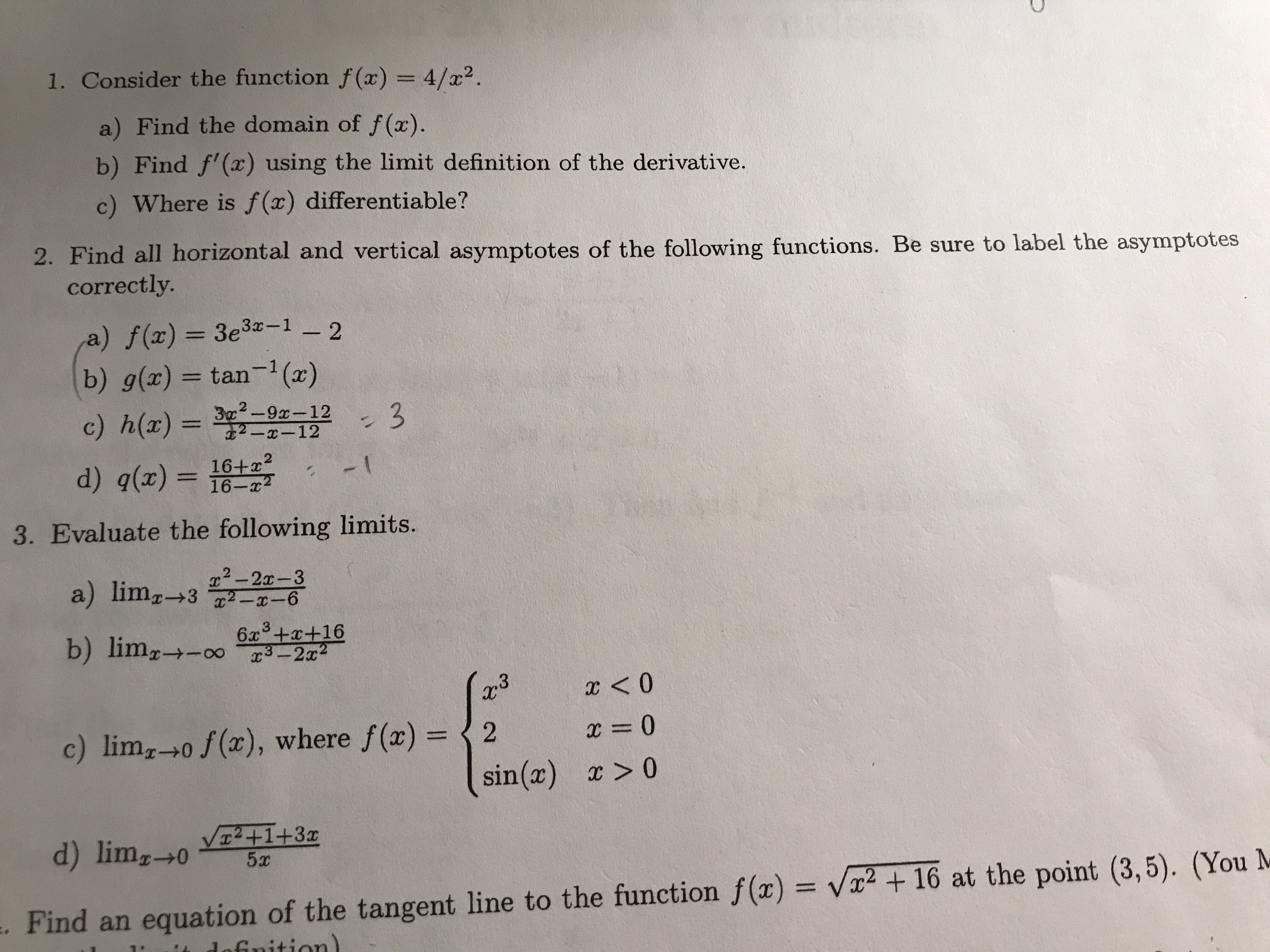# I. Consider the function f(x) 4/x2. a) Find the domain of f(x). b) Find f'() using the limit definition of the derivative. c) Where is f(x) differentiable? 2. Find all horizontal and vertical asymptotes of the following functions. Be sure to label the asymptotes correctly a) ()33z-1 2 (b) g(x) = tan-1 (2) c) h(x)-T2-92-112-3 --12 1 3. Evaluate the following limits. 2-2x-3 b) lim,ーナー0。6a3+2+16 c) lim,--0 f(x) , where f(x)-(2 x = 0 0 sin(x) V2+1+3c d) limx-W--5x . Find an equation of the tangent line to the function f(x) = Va2+16 at the point (3,5). (You N

Question

Can you help me answer question 2?help_outlineImage TranscriptioncloseI. Consider the function f(x) 4/x2. a) Find the domain of f(x). b) Find f'() using the limit definition of the derivative. c) Where is f(x) differentiable? 2. Find all horizontal and vertical asymptotes of the following functions. Be sure to label the asymptotes correctly a) ()33z-1 2 (b) g(x) = tan-1 (2) c) h(x)-T2-92-112-3 --12 1 3. Evaluate the following limits. 2-2x-3 b) lim,ーナー0。6a3+2+16 c) lim,--0 f(x) , where f(x)-(2 x = 0 0 sin(x) V2+1+3c d) limx-W--5x . Find an equation of the tangent line to the function f(x) = Va2+16 at the point (3,5). (You N fullscreen

## Expert Answer

### Want to see this answer and more?

Experts are waiting 24/7 to provide step-by-step solutions in as fast as 30 minutes!*

*Response times vary by subject and question complexity. Median response time is 34 minutes and may be longer for new subjects.
Tagged in© 2021 bartleby. All Rights Reserved.